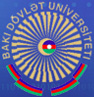## FD 2.17 Dissertation Council

ABSTRACTS

 TOPICS SPECIALTY FIELD OF SCIENCE APPLICANT Study of bulling out and release of energy of a three-layer piezoelectric+metal+piezoelectric circular plate with interlayer cracks 2002.01 - Mechanics of deformable solid Mathematics Fazila Ilyas kizi Cafarova On geometry of special riemannian metrics in differentiable manifolds and their bundles 1204.01 – Geometry Mathematics Sevil Farhat kizi Kazimova LIE algebroids and groupoids and properties of functors in the fuzzy modulus category 1210.01 – Topology Mathematics Sabuhi Eldar oglu Abdullayev Waves in a deformable pipe filled with viscous bubbly liquid 2003.01 – Fluid, gas and plasma Mathematics Reyhan Sayyad kizi Akbarly MATHEMATICAL MODELING OF THE SEALING PROCESS WITH SEALING ELEMENT 2002.01 - Mechanics of deformable solid Mathematics Kaklik Oruc kizi Rustamov HOMOLOGIES OF FUZZY MODULES 1201.01 – Algebra Mathematics Kamala Mutalib kizi Veliyeva STUDYING OF OPTIMAL CONTROL PROBLEMS WITH NON-LOCAL INTEGRO-DIFFERENTIAL EQUATIONS 1211.01 – Differential equations Mathematics Farah Mammad qizi Zeynalli STUDYING STRESS STRAIN STATE OF A SMALL THICKNESS INHOMOGENEOUS SPHERE 2002.01 – Mechanics of deformable solid Mathematics Natavan Sabir kizi Hasanova DISCRETE ADDITIVE, MULTIPLICATIVE AND POVERATIVE DERIVATIVE CONCEPTS AND APPLICATIONS TO SOLUTION OF THE CAUCHY AND BOUNDARY VALUE PROBLEMS FOR DIFFERENTIAL DERIVATIVE EQUATIONS 1211.01 – Differential equations Mathematics Aygun Malik Mammadzade FACTORIZATION METHOD TO DETERMINATE THE FUNDAMETAL SOUTIONS OF THE FRACTIONAL ORDER ORDINARY AND PARTIAL DIFFERENTIAL EQUATIONS 1211.01 – Differential equations Mathematics Allahshukur Azizaga Guliyev
 Mathematics